Constructing a triangle when 3 sides known (SSS)

Practical Geometry
Concept wise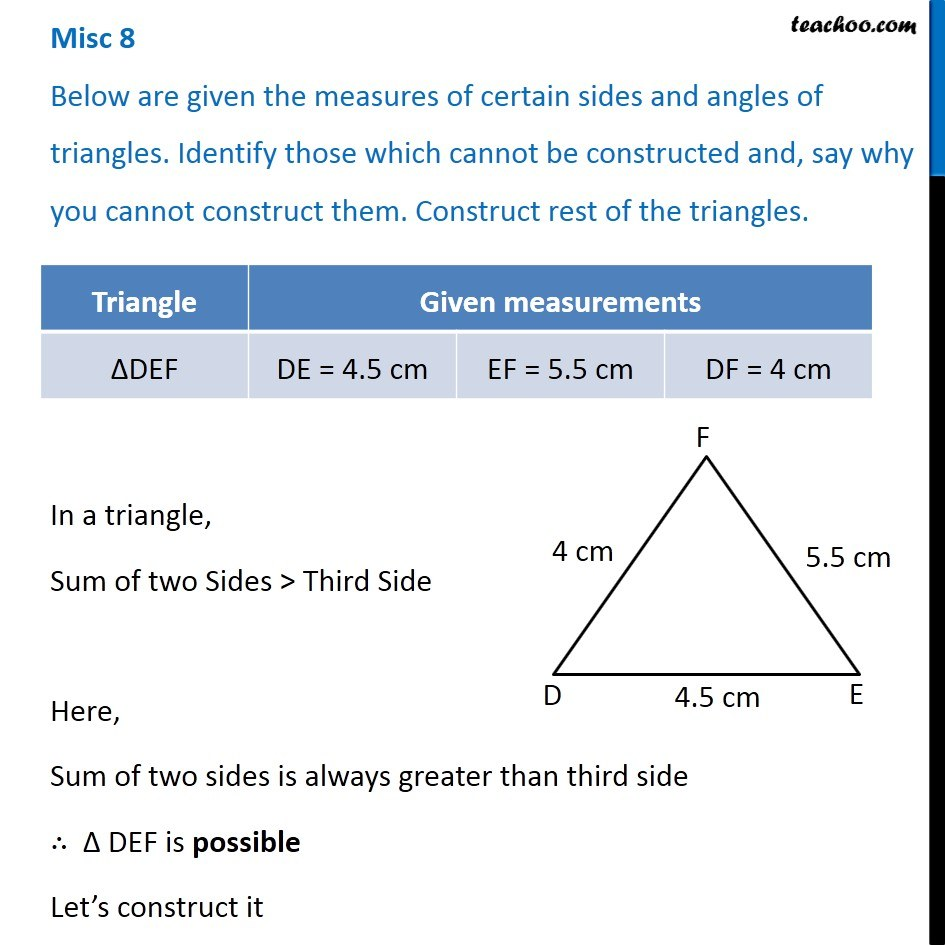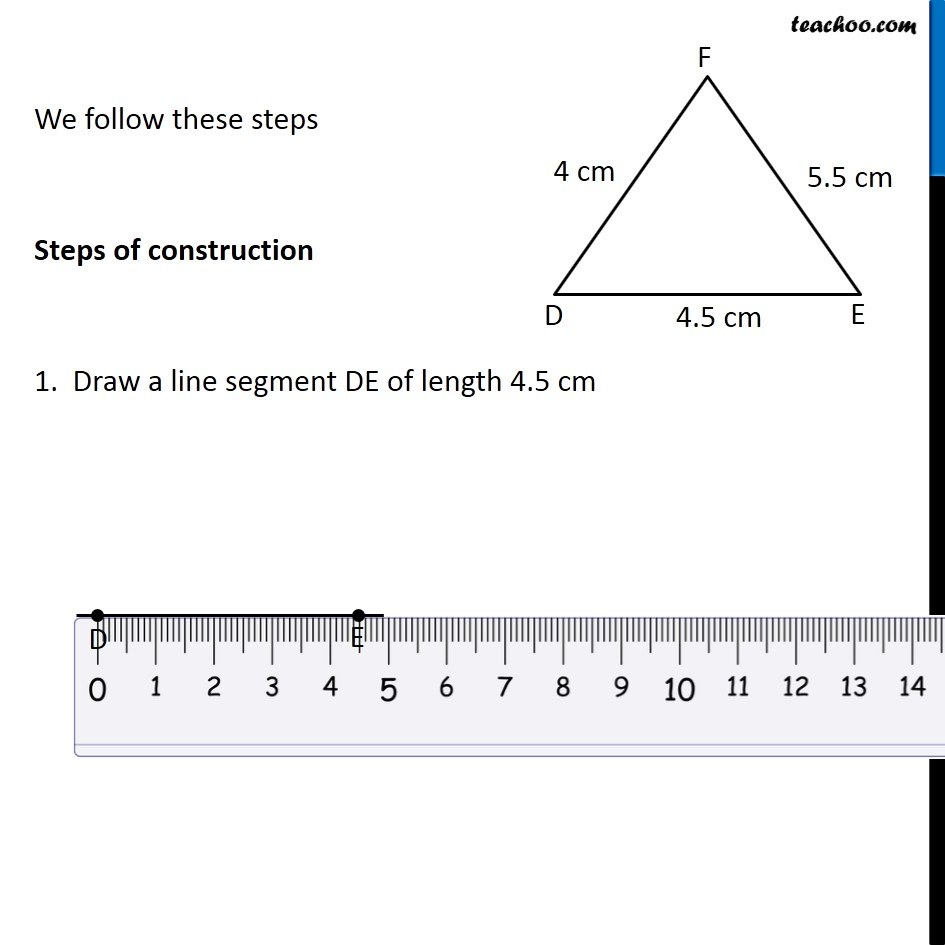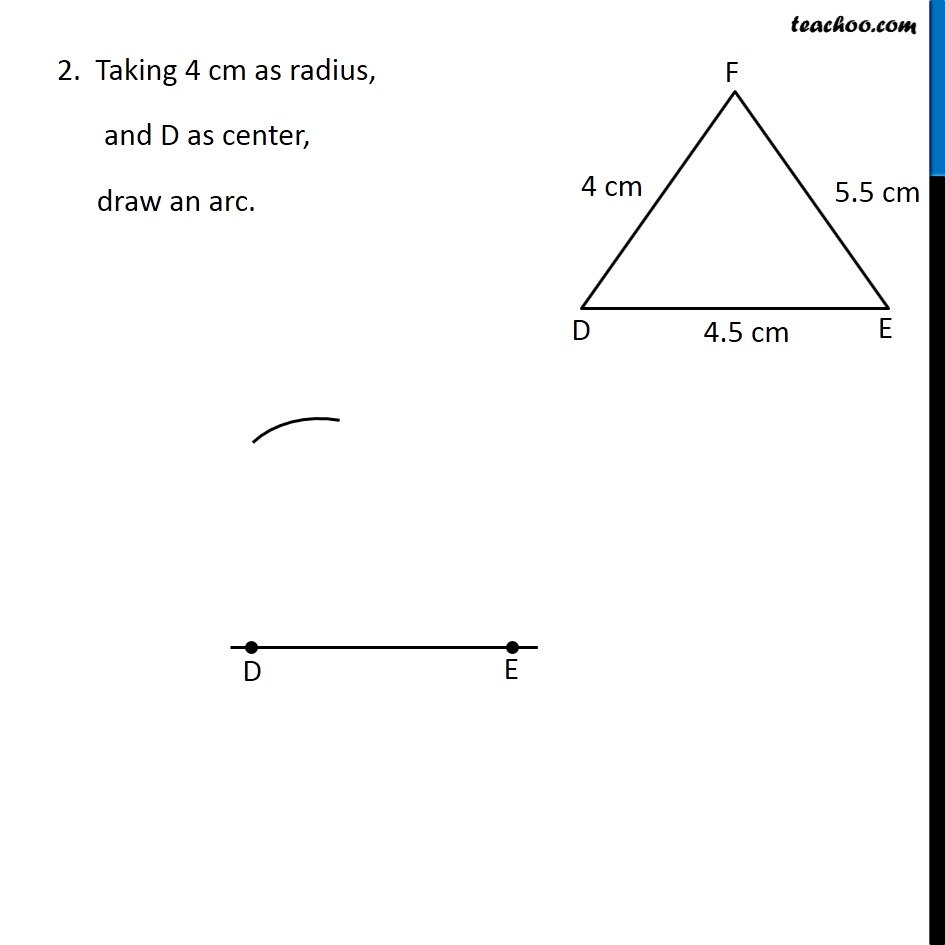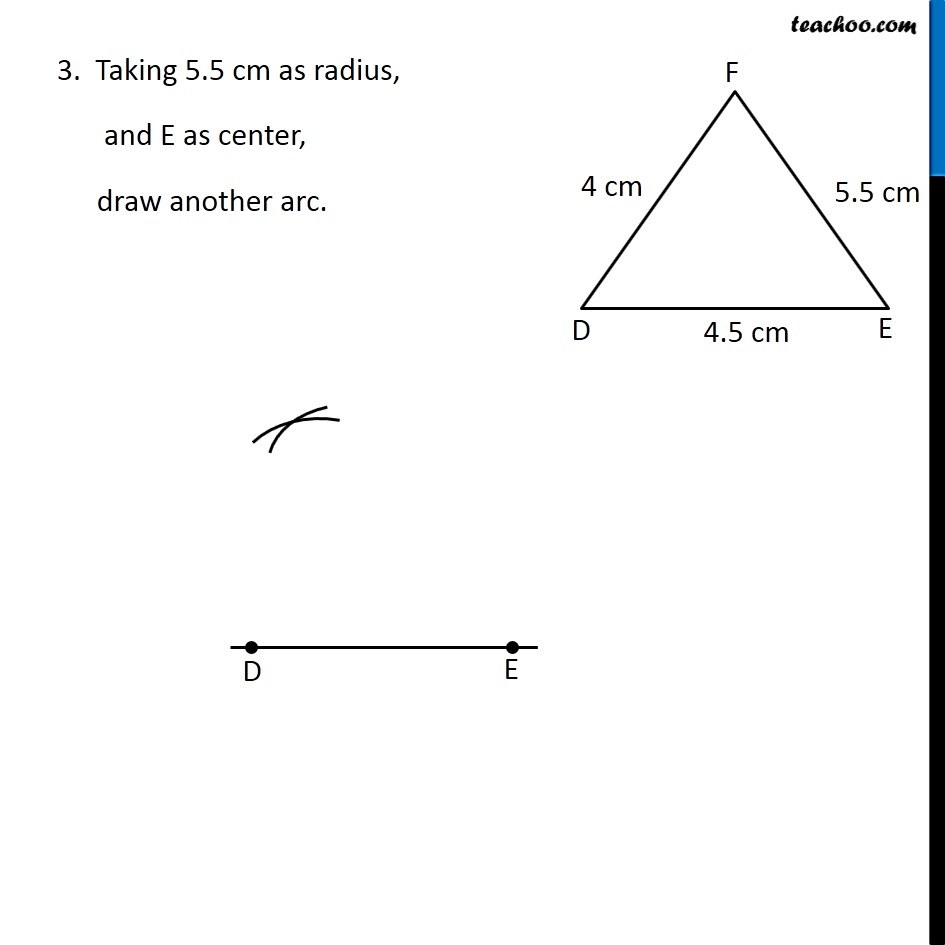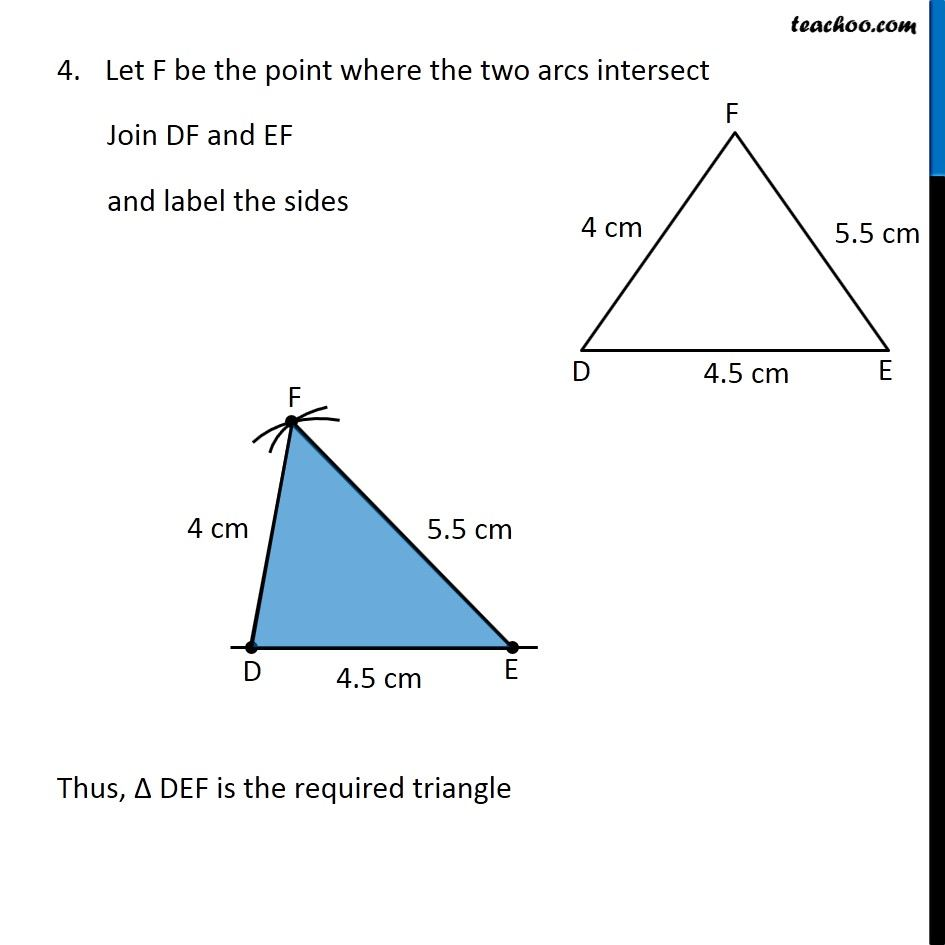Learn in your speed, with individual attention - Teachoo Maths 1-on-1 Class

### Transcript

Misc 8 Below are given the measures of certain sides and angles of triangles. Identify those which cannot be constructed and, say why you cannot construct them. Construct rest of the triangles. In a triangle, Sum of two Sides > Third Side Here, Sum of two sides is always greater than third side ∴ Δ DEF is possible Let’s construct it We follow these steps Steps of construction 1. Draw a line segment DE of length 4.5 cm 2. Taking 4 cm as radius, and D as center, draw an arc. 3. Taking 5.5 cm as radius, and E as center, draw another arc. Let F be the point where the two arcs intersect Join DF and EF and label the sides Thus, Δ DEF is the required triangle